# GRE Subject Test: Physics : Lenses

## Example Questions

### Example Question #1 : Optics

The focal length of a thin convex lens is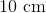. A candle is placed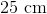to the left of the lens. Approximately where is the image of the candle?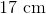to the right of the lens

No image is created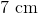to the right of the lensto the left of the lensto the left of the lensto the right of the lens

Explanation:

Because the object is beyond 2 focal lengths of the lens, the image must be between 1 and 2 focal lengths on the opposite side. Therefore, the image must be between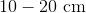on the right side of the lens.

Alternatively, one can apply the thin lens equation: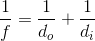Where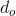is the object distance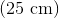andis the focal length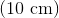. Plug in these values and solve.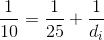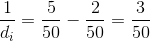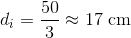### Example Question #1 : Lenses

A candle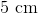tall is placedto the left of a thin convex lens with focal length. What is the height and orientation of the image created?

No image is created.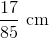, inverted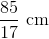, inverted, upright, upright, inverted

Explanation:

First, find the image distance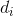from the thin lens equation: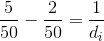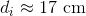Magnification of a lens is given by: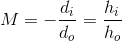Where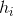and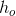are the image height and object height, respectively. The given object height is, which we can use to solve for the image height: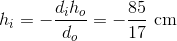Because the sign is negative, the image is inverted.

### Example Question #1 : Optics

Sirius is a binary star system, consisting of two white dwarfs with an angular separation of 3 arcseconds. What is the approximate minimum diameter lens needed to resolve the two stars in Sirius for an observation at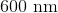?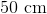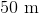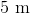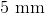Explanation:

The Rayleigh Criterion gives the diffraction limit on resolution of a particular lens at a particular wavelength: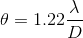Where theta is the angular resolution in radians,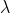is the wavelength of light, andis the diameter the lens in question. Solving forand converting 3 arcseconds into radians, one can approximate the diameter to be about: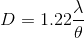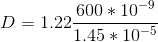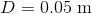### All GRE Subject Test: Physics Resources